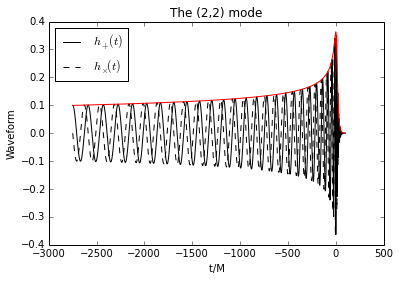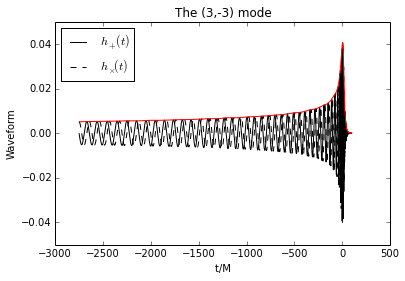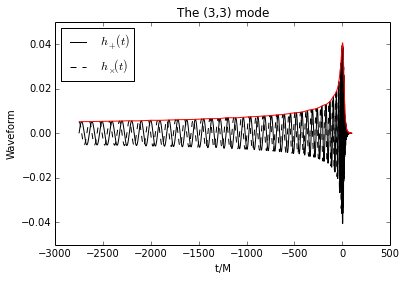Import GWSurrogate and other useful modules:

In :
import gwsurrogate as gws # import gwsurrogate package
import gwtools  # import gwtools (a collection of useful python functions included with gwsurrogate)

%matplotlib inline
import numpy as np, matplotlib.pyplot as plt


One can load only some modes of the surrogate data or all modes. Here, the (2,2) and (3,3) mode data is loaded from the full surrogate file.

In :
# Surrogate data located at http://www.black-holes.org/surrogates/
spec = gws.EvaluateSurrogate('SpEC_q1_10_NoSpin_nu5thDegPoly_exclude_2_0.h5', ell_m=[(2,2), (3,3)])

loading surrogate mode... l2_m2
loading surrogate mode... l3_m3


Generating a waveform mode is easy. For example, the (2,2) mode produced by a binary black hole coalescence with mass ratio 1.7 is:

In :
modes, times, hp, hc = spec(q=1.7, ell=, m=, mode_sum=False, fake_neg_modes=False)

# Plot the (2,2) mode
gwtools.plot_pretty(times, [hp, hc],fignum=1)
plt.plot(times,gwtools.amp(hp+1j*hc),'r')
plt.title('The (%i,%i) mode'%(modes,modes))
plt.xlabel('t/M ')
plt.show()Other waveform harmonics are easy to generate as well. For example, the (3,3) and (3,-3) modes produced by the same binary are:

In :
modes, times, hp, hc = spec(q=1.7, ell=, m=, mode_sum=False)

# Plot the (3,3) and (3,-3) modes
gwtools.plot_pretty(times, [hp[:,0], hc[:,0]],fignum=2)
plt.plot(times,gwtools.amp(hp[:,0]+1j*hc[:,0]),'r')
plt.xlabel('t/M ')
plt.title('The (%i,%i) mode'%(modes,modes))

gwtools.plot_pretty(times, [hp[:,1], hc[:,1]],fignum=3)
plt.plot(times,gwtools.amp(hp[:,1]+1j*hc[:,1]),'r')
plt.title('The (%i,%i) mode'%(modes,modes))
plt.xlabel('t/M ')
plt.show()All modes can be generated by default when excluding the ell and m options. All requested modes can be summed with their respective spin-weighted -2 spherical harmonics by default (mode_sum=True).

See the GWSurrogate documentation and IPython notebook tutorials for more features. GWSurrogate can be downloaded from https://pypi.python.org/pypi/gwsurrogate/.